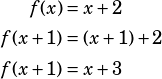HI!
I have a quick question on one of the practice questions. There was an explanation offered, but I’m still confused 🙁

This is for PWN 4th Edition –> Page 100, Question 2
f(x-1) = X +1 for all values of x, which of the following is equal to f(x+1)?
The explanation in the back of the book is a bit unclear to me and I really need another way to look at this problem Any help is appreciated, thanks in advance!

Remember that one helpful way to think about functions is that they’re like a machine (in the book I use the example of the car wash) that performs the same operation on anything that gets put into it. So if you know that the functionis defined as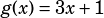, then you know that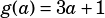. You also know that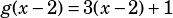, you know that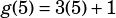, etc. In plain English, you know that whatever gets put into the functiongets multiplied by 3, and then 1 is added to the result.

In this question, we don’t know what the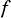function is, but we do know that when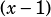gets put into the function,comes out. So the question we need to answer is really this: what arithmetic operations can the function be performing onto turn it into?

There’s nothing the function can divide by or multiply by to get that result. There are no exponents that would produce that result.

However, there is a simple addition the function could be doing to produce that result!

What if the function is simply adding 2? That would certainly turninto:.

If the function is just adding 2, then we can write the regularthusly: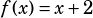. That means that whatever gets put into the function machine, 2 is added to it and nothing else happens.

Now, let’s use the definition we just figured out forto evaluate.